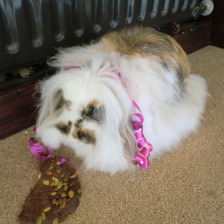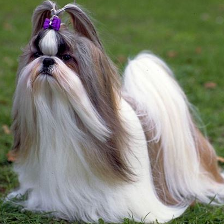# Classifying images with MobileNetV2

## MobileNetV2

Keras includes a number of pretrained networks ('applications') that you can download and use straight away. One of these is MobileNetV2, which has been trained to classify images.

You can simply import `MobileNetV2` from `keras.applications` and create an instance of it. The first time that you do this, the network will be downloaded from the internet.

If you don't pass any keywords to `MobileNetV2()`, then the network will have random weights; that is, you will get the architecture of the network, but not the weights, and you will therefore have to train it yourself. By specifying `weights='imagenet'`, you indicate that you want the network to be pretrained.

``````from keras.applications.mobilenet_v2 import MobileNetV2

model = MobileNetV2(weights='imagenet')
# model.summary()  # Uncomment this to print a long summary!
``````

## Preparing an image for model input

We're going to ask MobileNetV2 to which category the following image belongs:Figure 1. The Boef on her birthday.

This is a photo of The Boef when she was celebrating her birthday at my mother's place, where it seems that things escalated. The Boef is a bunny, but to a naive observer this may not be obvious. So it's interesting to see what MobileNetV2 sees in this photo.

Neural networks are picky when it comes to the kind of input that they expect. If you provide input in a format that the network doesn't expect, then the predictions won't make sense (if the structure of the input is superficially compatible with the network) or the code will simply crash (if the structure of the input is incompatible).

MobileNetV2 expects images of 224 × 224 pixels with three color channels. In other words, it expects input of shape `(224, 224, 3)`. You can pass multiple images at once to the model, just like we passed multiple numbers to our model in the previous tutorial. This means that the input should actually consist of four dimensions, where the first dimension corresponds to the index of the image.

Here we only pass a single image to the model, which means that the first dimension of the input is of size 1.

``````import numpy as np

data = np.empty((1, 224, 224, 3))
``````

When you load an image with `imread()`, the pixel values are in the 0 - 255 range. However, MobileNetV2 expects pixel values to be in the -1 to 1 range. You could perform this transformation yourself, but Keras also provides preprocessing functions:

``````from keras.applications.mobilenet_v2 import preprocess_input

data = preprocess_input(data)
``````

## Classifying an image

Now that our image is in the correct format for MobileNetV2, we can get the predictions. (See this previous tutorial if you're unsure how this works.)

``````predictions = model.predict(data)
print('Shape: {}'.format(predictions.shape))
``````

Output:

``````Shape: (1, 1000)
``````

As before, the predictions are returned as a two-dimensional array where the first axis corresponds to the observations (images, in this case). Since we have fed only a single image into the model, the first dimension is of size 1. The second axis corresponds to the output neurons of the model. Since MobileNetV2 has 1000 neurons in the output layer, the second dimension is of size 1000.

We can check which output neuron was most active using `np.argmax()`, which provides the index of the highest value:

``````output_neuron = np.argmax(predictions)
print('Most active neuron: {} ({:.2f}%)'.format(
output_neuron,
100 * predictions[output_neuron]
))
``````

Output:

``````Most active neuron: 155 (82.17%)
``````

However, knowing that neuron 155 became the most active in response to the image of The Boef is not, by itself, very informative, because we also need to know which category this corresponds to. Fortunately, Keras provides a function that automatically maps predictions onto scores for the corresponding categories:

``````from keras.applications.mobilenet_v2 import decode_predictions

for name, desc, score in decode_predictions(predictions):
print('- {} ({:.2f}%%)'.format(desc, 100 * score))
``````

Output:

``````- Shih-Tzu (82.17%%)
- Pekinese (8.71%%)
- Lhasa (3.47%%)
- Tibetan_terrier (0.77%%)
- Old_English_sheepdog (0.28%%)
``````

So MobileNetV2 is fairly confident that The Boef is a Shih-Tzu. Fair enough, MobileNetV2.Figure 2. A Shih-Tzu.

## Video tutorial

Video 1. Watch this tutorial on YouTube!

You're done with this section!